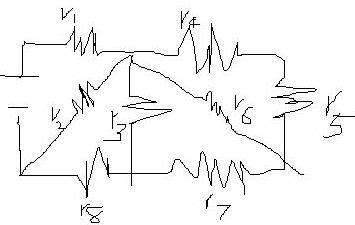# Find the total resistance

• I_am_Atomos
In summary, this conversation was about how to solve for the total resistance of a circuit with multiple resistors. The original problem was to find the total resistance of a circuit with four resistors, but one of the resistors was replaced by a single resistor. The solution was found to be incorrect, but the starting point was given.f

## Homework Statement

Find the total resistance of this circuit:each resistance in this circuit is 20ohms.
(r1=r2=r3=r4=r5=r6=r7=r8=20ohms)

## Homework Equations

Rt=r1+r2+r3... for series
1/Rt=1/r1+1/2r+1/r3... for parallel

## The Attempt at a Solution

since r4 and r5 is parallel to r6
so r4 to r6
= 1/(1/(20+20)+1/20)
=13.333333333333
r7 is in series with r4 to r6
r4 to r7
=13.333333333333+20
=33.333333333333
r3 is parallel with r4 to r7
r3 to r7
=1/(1/33.333333+1/20)
=12.5
r8 is in series with r3 to r7
r3 to r8
=12.5+20
=32.5
r2 is parallel with r3 to r8
r2 to r8
=1/(1/32.5+1/20)
=12.38
r1 is in series with r2 to r8
rt
=20+12.38
=32.38

yea i got it and i think it's right except my friend said it's wrong but i can't figure out where

Replace r4 to r6 by a single resistor since you solved for its resistance. Then check if that single resistor is in series with r7. Keep redrawing the pic with the simplified resistors and work your way to the left.

Replace r4 to r6 by a single resistor since you solved for its resistance. Then check if that single resistor is in series with r7. Keep redrawing the pic with the simplified resistors and work your way to the left.

isn't that what i did on my solution?

I will give you a starting point. The new resistor you found in your first step is not in series with r7. If you drew it properly in the schematic it would in fact be in parallel with r3 and r7.

Looks to me for the configuration shown like:

(((((R4 + R5) || R6) + R7) || R3) + R8) || R2) + R1

That comes out to 20 + 260/21 = 32.381 Ω

Edit: Nevermind, I looked at it again and see how R7 can be in series with the new resistor. It is like an optical illusion :)

So what you did looks correct.

Alright, I will say what it looks to me:

((((((R4 + R5) || R6) || (R3 + R7)) + R8) || R2) + R1) = 32 ohms

Your equation looks to depend on R8 being connected to the far node, the junction of R5, R6, R7, and not the node between R3 and R7

Yeah, I was wrong. I have always been bad with mazes as well.